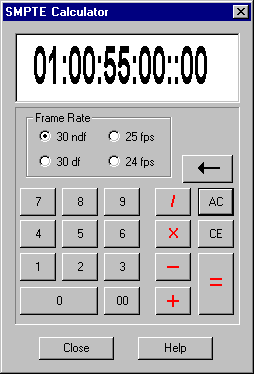# SMPTE Calculator#### What is the SMPTE Calculator?

The SMPTE Calculator is a tool to add, subtract, multiply and divide SMPTE values.

#### How does it work?

The SMPTE Calculator works very much like any other calculator, but it works with SMPTE numbers instead. A SMPTE number is divided into hours, minutes, seconds, frames and subframes. The calculator can also handle 4 different types of SMPTE code: 30 non-drop frame, 30 drop frame, 25 frames per second and 24 frames per second.

You may change the SMPTE frame rate at any time, and the current SMPTE values will be converted to the new frame rate. The way this calculator works is, it converts absolute subframe values when converting frame rates. So if you have 01:00:00:00::00 at 30ndf and convert it to 25fps, then the calculator converts the value internally to a total subframe count (108000 x subframes per frame) this value is then converted from a total subframe count to the new SMPTE type: 01:12:00:00 at 25fps.

The calculator will use the same subframes per frame as selected in the Special preferences (either 80 or 100).

#### Entering values

You don’t have to enter the colons between values – as numbers are entered into the calculator, they move from right to left and drop off the left side of the SMPTE field. Similarly, when the calculator requires a floating-point value for multiplication and division, you don’t have to enter the decimal point.

Floating-point values are limited to a maximum of 999.99

You can also click directly on the SMPTE window and enter values with the keyboard and use the arrow keys to change SMPTE values.

#### The Keyboard

You may enter values with the keyboard or the mouse. The following keys perform special functions:

`·          A                  All Clear`
`·          C                  Clear Entry`
`·          .(period)          00 (double zero)`
`·          Enter              Equals`